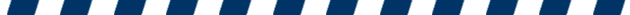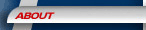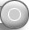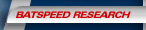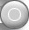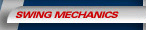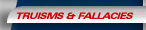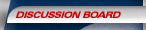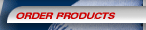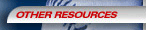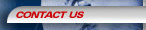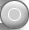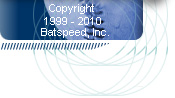Re: Re: Re: Re: Resolving Conflict

Posted by: Mike Myers (mike.myers@bell.ca) on Mon Oct 25 09:55:56 2004

>>> Ok. But in your dragster post, you indicate:
>
> "Bonds and Sosa may only attain 3 or 4 mph bat-head velocity during pre-launch torque. But this does not mean their contact velocity is only increased by 3 or 4 mph."
>
> Given force is constant, isn't this exactly what it means?
>
> If so, then have we not demonstrated pre-launch torque cannot contribute significantly to overall batspeed?
>
> (Thanks for discussion Jack - just trying to resolve logic.) <<<
>
> Hi Mike
>
> I thank you for your helpful input.  It may seem that having the bat moving at slow pace would add little to the contact bat speed. But, I would not suggest to Bonds or Sheffield that they could hit a ball just as hard starting with a static bat. Do you think allowing a runner in a 60 yard dash a running start of 3 or 4 mph would have any significance on the outcome of the race?
>
> Jack Mankin
>
>
>

Jack,

Of course it would. But the outcome of a 60 yard dash is a function of position wrt time, not velocity wrt time.

Known:
- 60 yard (54.864m) dash
- 2 runners
- R1 starts at 4 mph (1.788m/s), R2 starts at 0 mph

Assumptions:
- R1 and R2 have identical mass
- constant force (and thus acceleration) on each runner
- R1 and R2 have acceleration a = 1 m/s^2

Then:
d = vi*t + (at^2)/2

For R1:
d=54.864m
vi=1.788m/s
a=1m/s^2

54.864 = 1.788*t + (1*t^2)/2
109.728 = 3.576*t + t^2
t^2 + 3.576*t - 109.728 = 0
t = 8.839s

vf^2 = vi^2 + 2ad
= 1.788^2 + 2*1*54.864
vf = 10.627m/s

For R2:
d=54.864m
vi=0
a=1m/s^2

54.864 = (1*t^2)/2
t^2 = 109.728
t = 10.475s

vf^2 = vi^2 + 2ad
= 0 + 2*1*54.864
vf = 10.475m/s

Conclusion:
There is a significant difference of 1.64s between the runners (15.7% of R2) to complete the race, but the difference in runner speed at the finish line is only 0.152m/s (1.45% of R2).

For maximal contact power, we are only concerned with final batspeed, not how much time it takes to complete the swing. One can swing through a given path much faster, and produce little more final batspeed.

Mike.

Followups:

Post a followup:
Name:
E-mail:
Subject:
Text:

Anti-Spambot Question:What is the MLB championship called?    World Championship    World Series    The Finals    The Cup

[   SiteMap   ]